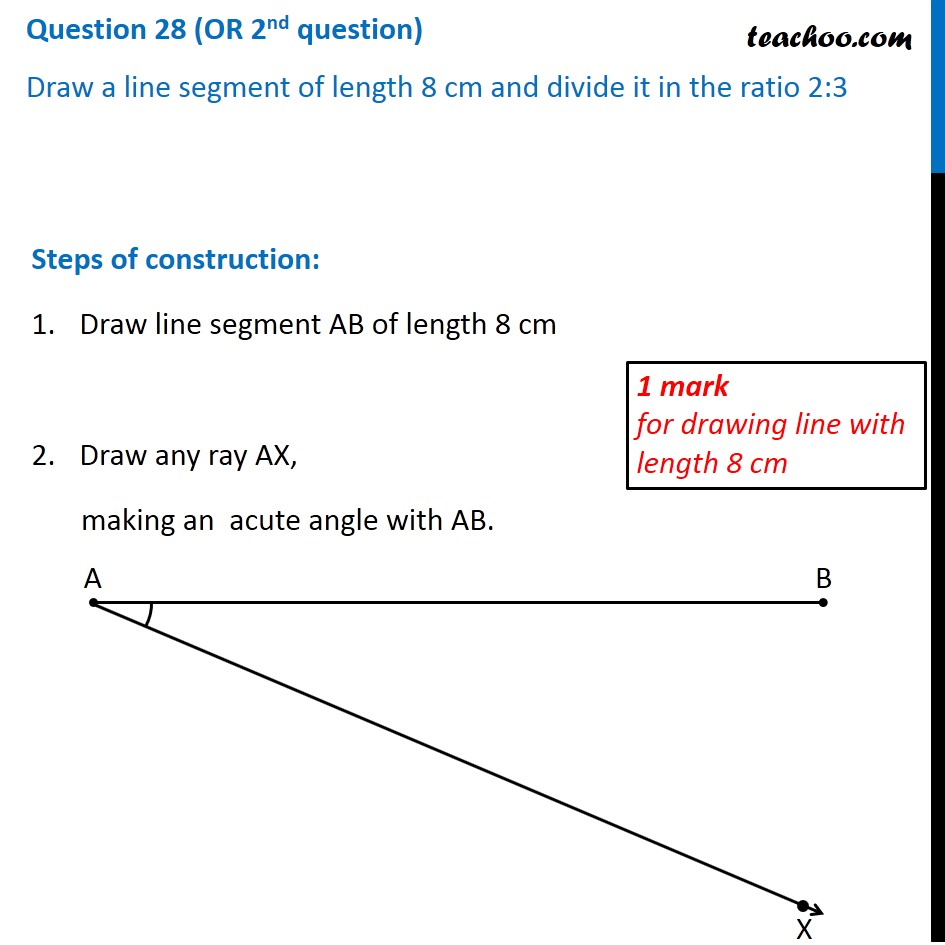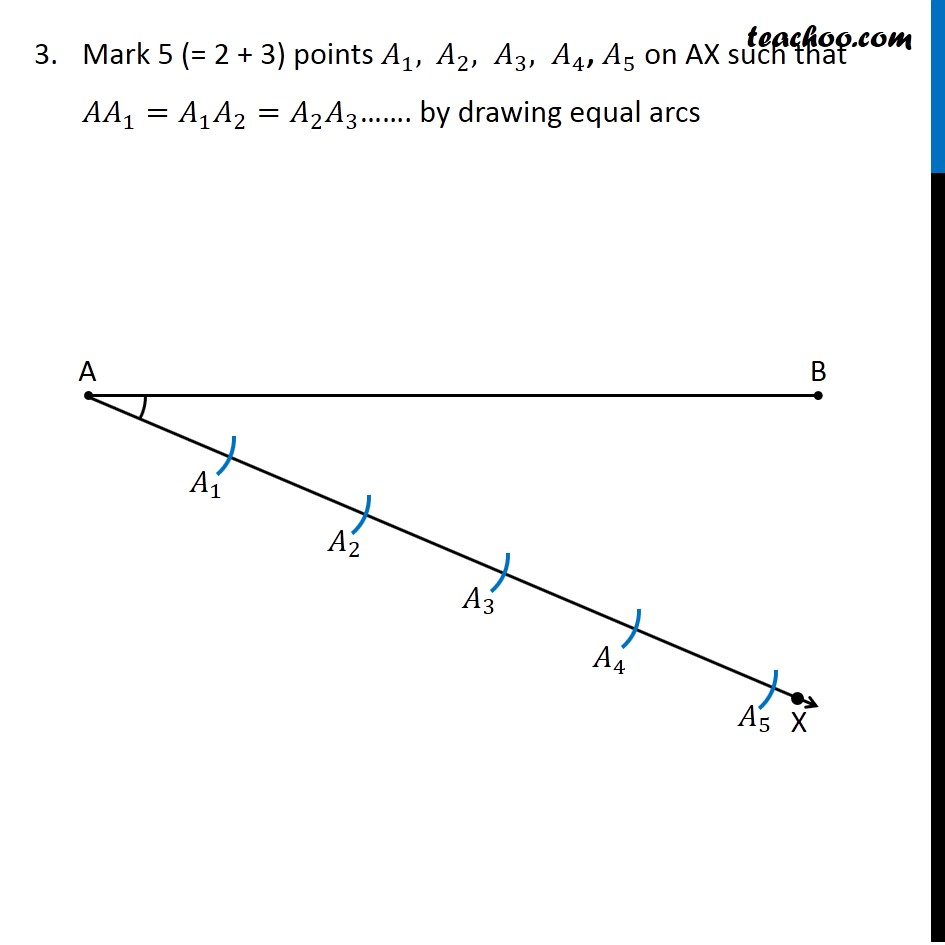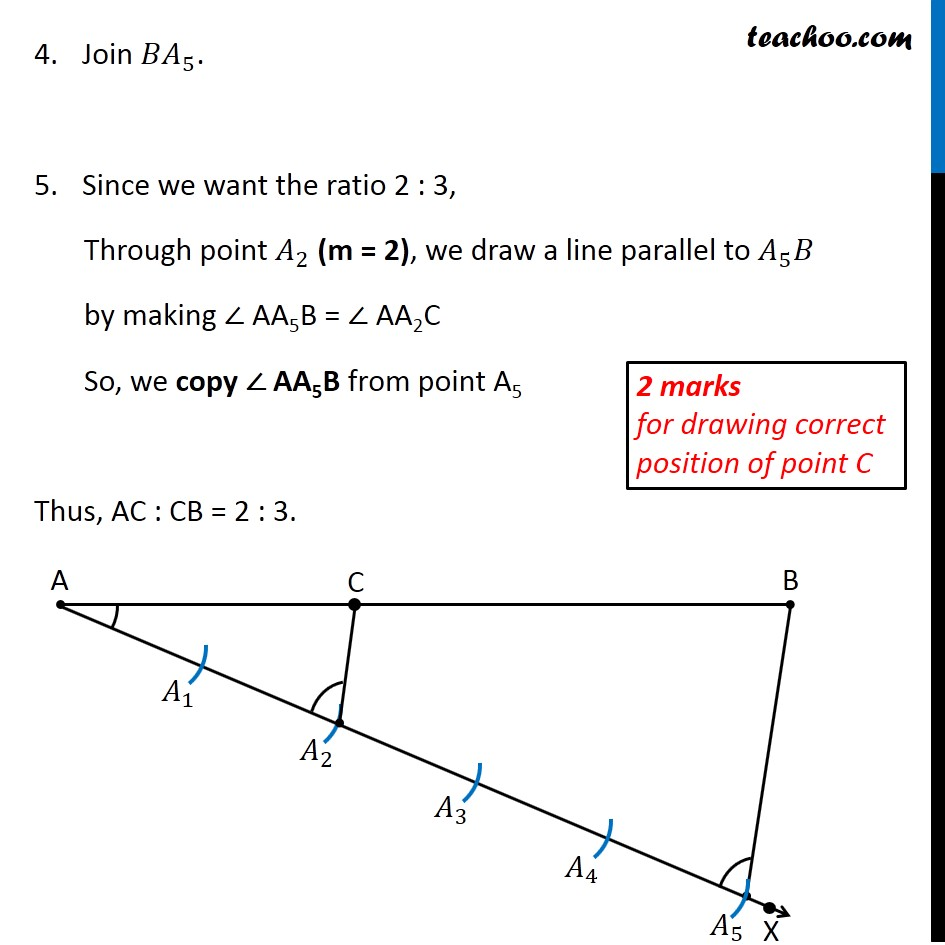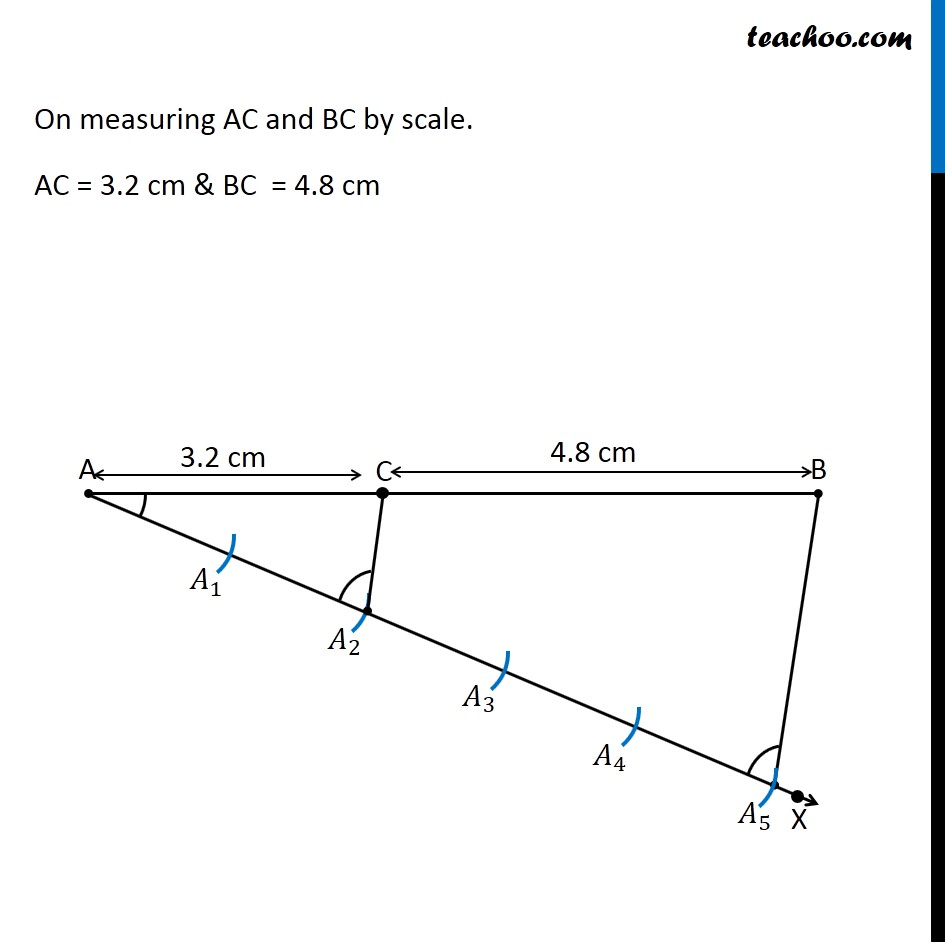CBSE Class 10 Sample Paper for 2020 Boards - Maths Basic

Class 10
Solutions of Sample Papers for Class 10 Boards

## Draw a line segment of length 8 cm and divide it in the ratio 2:3Note : This is similar to Ex 11.1, 1 of NCERT – Chapter 11 Class 10

Learn in your speed, with individual attention - Teachoo Maths 1-on-1 Class

### Transcript

Question 28 (OR 2nd question) Draw a line segment of length 8 cm and divide it in the ratio 2:3 Steps of construction: Draw line segment AB of length 8 cm Draw any ray AX, making an acute angle with AB. Mark 5 (= 2 + 3) points 𝐴_1, 〖 𝐴〗_2, 𝐴_3, 𝐴_4, 𝐴_5 on AX such that 〖𝐴𝐴〗_1=𝐴_1 𝐴_2=𝐴_2 𝐴_3……. by drawing equal arcs Join 〖𝐵𝐴〗_5. Since we want the ratio 2 : 3, Through point 𝐴_2 (m = 2), we draw a line parallel to 𝐴_5 𝐵 by making ∠ AA5B = ∠ AA2C So, we copy ∠ AA5B from point A5 Thus, AC : CB = 2 : 3. On measuring AC and BC by scale. AC = 3.2 cm & BC = 4.8 cm